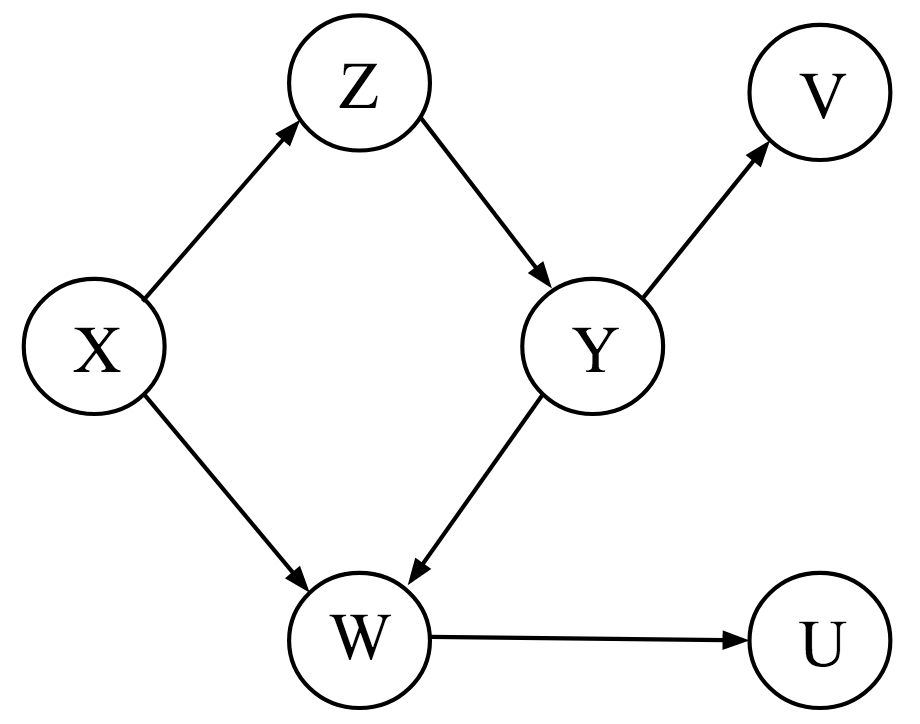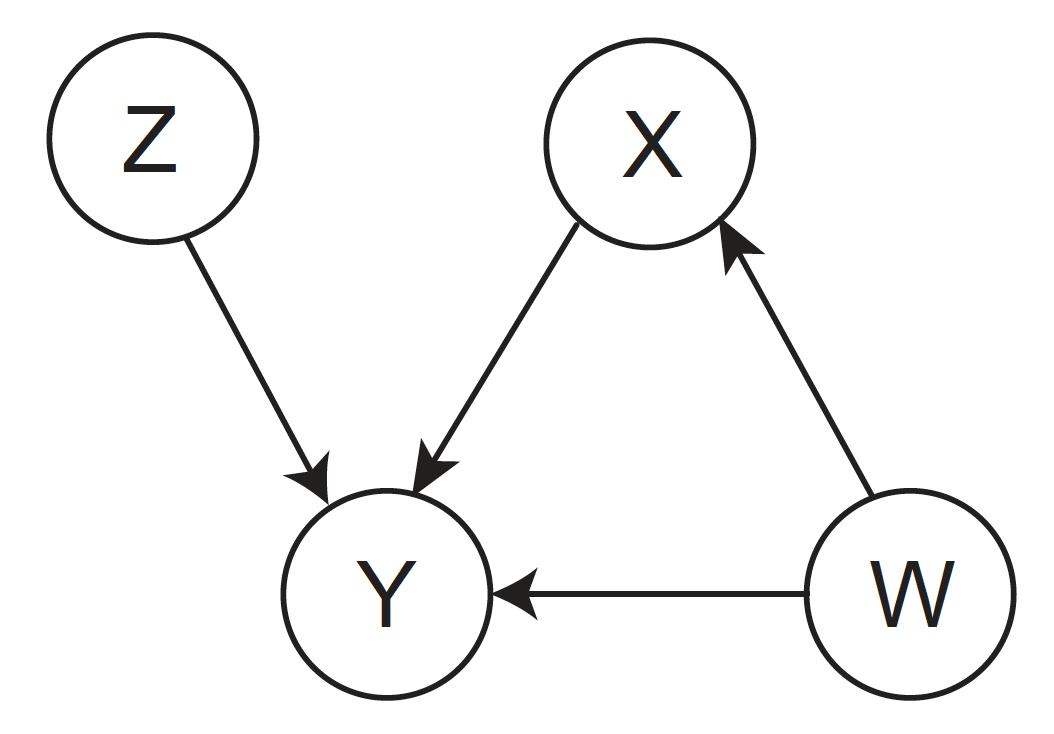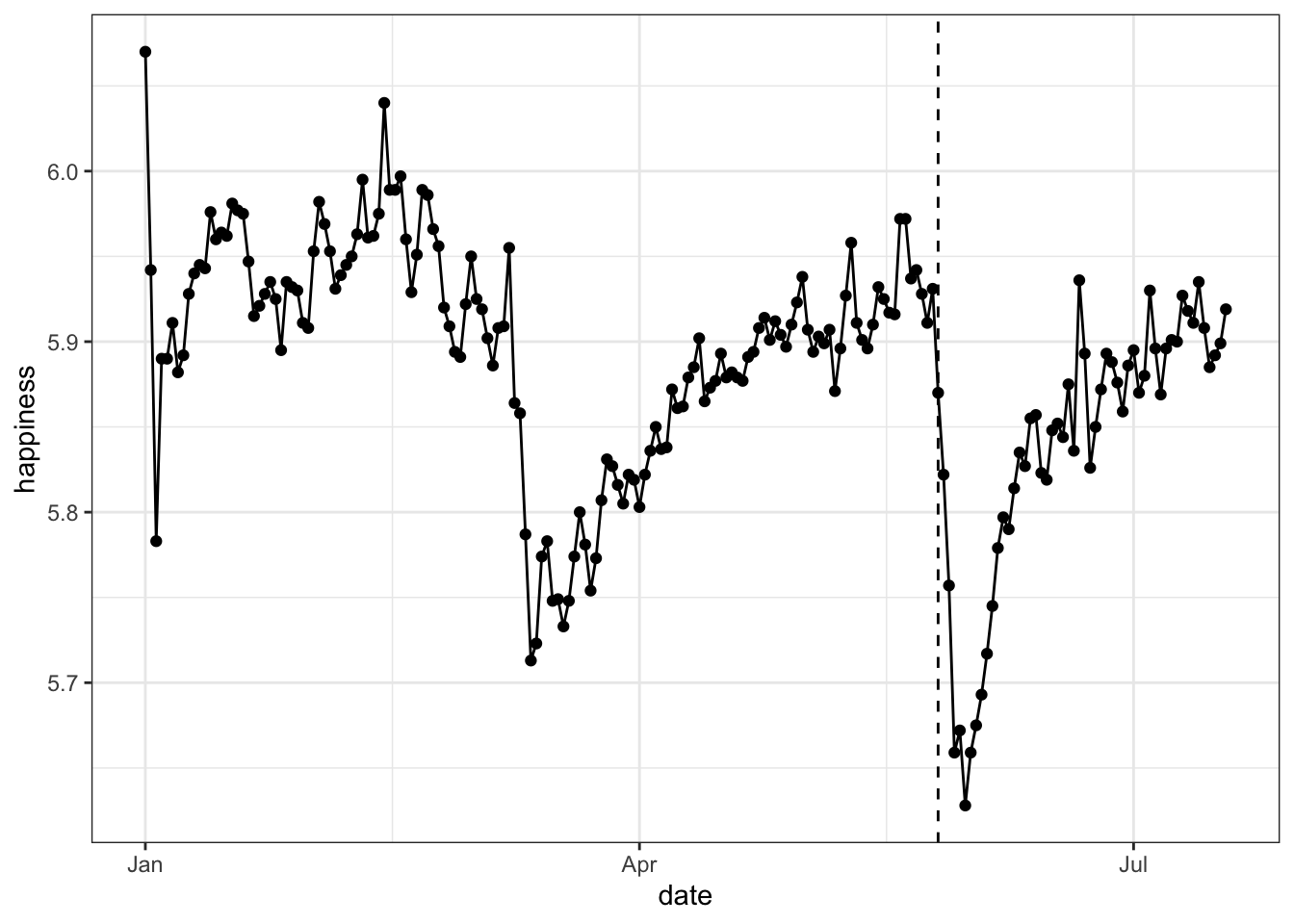# Exercise block I

## Marginal and conditional independencies

List all marginal and conditional independencies shown in the DAG below. (Note that conditional independence is symmetric, i.e., if $$X \perp Y \mid Z$$ then $$Y \perp X \mid Z$$).## A medical emergency

Below is a data set about kidney stones $$S \in \{\text{small}, \text{large}\}$$, medical treatments $$T \in \{A, B\}$$, and recovery $$R \in \{0, 1\}$$, where $$R = 1$$ indicates recovery. The size of the kidney stone acts as a common cause, influencing the medical treatment as well as the outcome.

$\begin{array}{r|c|c} & \text{Treatment A} & \text{Treatment B}\\\hline \text{Small Stones } { \left(\frac{357}{700} = 0.51\right)}& \frac{81}{87} = {0.93} & \frac{234}{270} = 0.87\\\hline \text{Large Stones } { \left(\frac{343}{700} = 0.49\right)} & \frac{192}{263} = {0.73} & \frac{55}{80} = 0.69\\\hline & \frac{273}{350} = 0.78 & \frac{289}{350} = {0.83} \end{array}$

• Draw the DAG.
• Compute $$P(R = 1 \mid do(T = A))$$ and $$P(R = 1 \mid do(T = B))$$. Which treatment is better?

## DAGs, $$d$$-separation, and causal effects

### Drawing a DAG

• Draw the DAG induced by this SCM:

\begin{aligned} S &:= \epsilon_S \\[.5em] T &:= S + \epsilon_T \\[.5em] U &:= W + \epsilon_U \\[.5em] V &:= 0.90 Z + \epsilon_V \\[.5em] W &:= 0.50 S + 1 X + 3 Y + \epsilon_W \\[.5em] X &:= Z + \epsilon_X \\[.5em] Y &:= 1.50V + X + \epsilon_Y \\[.5em] Z &:= \epsilon_Z \end{aligned}

• where

$(\epsilon_S, \epsilon_T, \epsilon_U, \epsilon_V, \epsilon_W, \epsilon_X, \epsilon_Y, \epsilon_Z) \stackrel{iid}{\sim} \mathcal{N}(0, 1)$

### Applying $$d$$-separation

• Using the previously drawn DAG, mark the following statement as either correct or incorrect:
• Regressing $$T$$ onto $$Y$$ yields no effect (Note: $$T$$ onto $$Y$$ means ‘lm(t ~ y)’)
• Regressing $$T$$ onto $$Y$$ while adjusting for $$W$$ yields no effect
• $$W$$ and $$T$$ are marginally independent
• $$Y$$ is a collider on the path from $$X$$ to $$U$$ that goes through $$Y$$
• $$Y$$ is independent of $$Z$$ given given $$X$$ and $$V$$
• $$S$$ is independent of $$Y$$ given $$U$$
• $$T$$ and $$U$$ are marginally independent
• $$T$$ and $$Y$$ are marginally independent
• $$X$$ and $$S$$ are marginally independent
• $$X$$ is independent of $$U$$ given $$W$$
• $$X$$ is independent of $$V$$ given $$Z$$
• $$X$$ is independent of $$V$$ given $$Z$$ and $$U$$
• Generate $$n = 2000$$ observations from the previous DAG
• Check your above answers by running the relevant regressions / conditional independence tests

### Causal effects and confounding

Use the previous DAG for these questions.

• Compute $$\mathbb{E}\left[W \mid X = x\right]$$
• Does it correspond to the causal quantity in the SCM? Why / why not?

• Compute $$\mathbb{E}\left[W \mid do(X = x)\right]$$

• What is the valid adjustment set to compute the average causal effect $$ACE(Z \rightarrow U)$$

• Visualize the marginal distribution of $$W$$ under the interventional DAG where $$Y:= 2$$
• Compare it with the marginal distribution under the observational DAG
• What do you observe? Why?

• Implement the intervention $$Y:= y$$ for $$y \in [0, 4]$$
• What do you expect $$\mathbb{E}\left[W \mid do(Y = y)\right]$$ will look like as a function of $$y$$? Why?
• What do you expect $$\text{Var}\left[W \mid do(Y = y)\right]$$ will look like as a function of $$y$$? Why?
• Visualize both!

• Visualize and analyze the data. What do you observe?
• Draw a DAG that could underlie these data. Which analysis is the correct one?

# Exercise block II

## PC Algorithm

### Pen and paper

Suppose you know that the true DAG is given by the following. Before using the PC algorithm on data generated from this DAG, go through the steps that the PC algorithm does by hand. What is the resulting CPDAG?### Using pcalg

Suppose that we have the following data.

library('pcalg')

n <- 1000

X <- rnorm(n)
W <- rnorm(n)
Z <- 0.50 * X + rnorm(n)
Q <- -0.75 * X + Z + W +  rnorm(n)

dat <- cbind(W, X, Z, Q)

fit <- ...
plot(fit, main = '')
• Use the pcalg package to estimate the DAG. Does this conclusion agree with what you find by doing the PC algorithm by hand?

### What’s wrong here?

Use the data set available from here, which follows the same DAG, and redo the procedure you did above. What do you observe?

• In case you find that the estimated DAGs are different, what would explain this?
• Can you recover the DAG in the previous exercise? (Hint: Take a look at the kpcalg package.)

## Who is my causal parent?

Note that $$X \sim \mathcal{N}(\mu, \sigma)$$ is the same as $$X = \mu + \sigma\varepsilon$$ where $$\varepsilon \sim \mathcal{N}(0, 1)$$. Suppose you observe the following SCM in environment $$e = 1$$:

\begin{aligned} X_1^1 &\sim \mathcal{N}(0, 1) \\[0.50em] X_2^1 &\sim \mathcal{N}(2, 0.50) \\[0.50em] Y^1 &\sim \mathcal{N}(X_2^1 - X_1^1, 1) \\[0.50em] X_3^1 &\sim \mathcal{N}(0.60 \, Y^1 + 0.80 \, X_2^1, 1) \enspace , \end{aligned}

and this one in environment $$e = 2$$:

\begin{aligned} X_1^2 &\sim \mathcal{N}(0, 1) \\[0.50em] X_2^2 &\sim \mathcal{N}(2, 2) \\[0.50em] Y^2 &\sim \mathcal{N}(X_2^2 - X_1^2, 1) \\[0.50em] X_3^2 &\sim \mathcal{N}(0.60 \, Y^2 + 0.80 \, X_2^2, 1) \enspace , \end{aligned}

and finally this one in $$e = 3$$:

\begin{aligned} X_1^3 &\sim \mathcal{N}(0, 1) \\[0.50em] X_2^3 &\sim \mathcal{N}(2, 0.50) \\[0.50em] Y^3 &\sim \mathcal{N}(5 + X_2^3 - X_1^3, 1) \\[0.50em] X_3^3 &\sim \mathcal{N}(0.60 \, Y^3 + 0.80 \, X_2^3, 1) \enspace . \end{aligned}

Suppose you observe $$n = 500$$ data points from all three SCMs.

• Use data from $$e = 1$$ and $$e = 2$$ and invariant causal prediction to find the causal parents of $$Y$$. What do you find?
• Include data from $$e = 3$$ as well. What happens? Why?

## $$X \rightarrow Y$$ or $$Y \rightarrow X$$?

Try to learn the causal direction for the following cause-effect pairs:

• Altitude and temperature
• Age and height
• $$\text{CO}_2$$-Emissions and energy use
• Employment and population

url <- 'https://webdav.tuebingen.mpg.de/cause-effect/'

temp <- read.table(paste0(url, 'pair0001.txt'), col.names = c('altitude', 'temperature'))
age <- read.table(paste0(url, 'pair0022.txt'), col.names = c('age', 'height'))
co2 <- read.table(paste0(url, 'pair0073.txt'), col.names = c('co2', 'energy'))
pop <- read.table(paste0(url, 'pair0084.txt'), col.names = c('employment', 'population'))

You need to restrict the Structural Causal Model to be able to do this. Compare the results of the Linear Non-Gaussian Acyclic Model (use the lingam function in the pcalg package) with the results of using a Nonlinear Gaussian Additive Noise Model (use the gam function in the mgcv package). Which method performs better? (Use the dhsic.test function from the dHSIC for independence tests).

Reproduce Alex Hayes’s analysis of the causal effect of Elon Musk’s tweet on the Tesla stock price using the CausalImpact package. The code below loads the data, but the use of riingo requires an account, which you probably don’t want to create. I’ve downloaded the data, which you can access from here. You may find this vignette useful.

library('riingo')
library('CausalImpact')

tesla <- riingo_iex_prices(
'TSLA', start_date = '2020-05-01', end_date = '2020-05-01', resample_frequency = '1min'
)

sp500 <- riingo_iex_prices(
'SPY', start_date = '2020-05-01', end_date = '2020-05-01', resample_frequency = '1min'
)

dat <-  data.frame(
'date' = tesla$date, 'tesla' = tesla$close,
'sp500' = sp500$close ) head(dat) ## date tesla sp500 ## 1 2020-05-01 13:30:00 762.440 285.400 ## 2 2020-05-01 13:31:00 759.200 285.560 ## 3 2020-05-01 13:32:00 759.990 285.850 ## 4 2020-05-01 13:33:00 764.850 285.780 ## 5 2020-05-01 13:34:00 771.565 285.950 ## 6 2020-05-01 13:35:00 769.570 285.405 • What is the estimate of the causal effect of Elon Musk’s tweet on the Tesla stock price? • In what sense does the answer depend on time? Do the model predictions make sense? • Suppose you had only hourly data, what problems would you run into when doing this analysis? • How confident are you in this causal effect estimate? What are potential problems? ## George Floyd’s death and global sadness In this exercise, you will use an interrupted time-series design to estimate the causal effect of the death of George Floyd on global happiness (as measured through tweets by hedonometer.org). You can get the Twitter data using the code below, which uses their API. library('httr') library('dplyr') library('ggplot2') library('jsonlite') res <- GET( 'http://hedonometer.org/api/v1/happiness/?format=json&timeseries__title=en_all&date__gte=2020-01-01&limit=200' ) dat <- fromJSON(rawToChar(res$content))$objects %>% mutate( date = as.Date(date), happiness = as.numeric(happiness) ) %>% arrange(date) George Floyd was murdered on the 26th of May. ix <- which(dat$date == '2020-05-26')

ggplot(dat, aes(x = date, y = happiness)) +
geom_line() +
geom_point() +
geom_vline(xintercept = as.Date('2020-05-26'), linetype = 'dashed') +
theme_bw()You can specify a custom time-series model that is being estimated on the happiness data before the death of George Floyd as follows.

happiness <- dat[, 3]
post_period <- seq(ix, nrow(dat)) # Period after death

happines_post <- happiness[post_period]
happiness[post_period] <- NA # Assume we don't have data after the intervention

# Custom model specification
impact <- CausalImpact(bsts.model = bsts.model, post.period.response = happines_post)